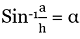# valarray asin() Function in C++ with Examples

C++ valarray asin() Function: Here, we will learn about the asin() function, its usages, syntax and examples.
Submitted by Shivang Yadav, on May 20, 2022

The valarray class in C++ is a special container that is used for holding elements like an array and performing operations on them.

Mathematical Arc Sine

Mathematically, arc sine is the inverse of sine function. The arc sine often known as sin inverse is used to evaluate the value of angle using the given ratio of side opposite to the angle and hypoteneuse.

Arc Sine is denoted as sin-1 and formulated as,Where,

• a is the length of side opposite to the angle
• h is the length of hypotenuse
• α is the angle

## std::asin(std::valarray) Function

The asin() function in valarray class is a function used for a valarray consisting of elements calculated as sin-1 for each element of the original valarray.

Syntax:

```template<class T> valarray<T> asin (const valarray<T>& x);
```

Parameter(s): It takes one parameter the valarray on which the mathematical operation is to be applied.

Return Value: It returns a valarray consisting of sin-1 value of each element of the initial valarray.

This is all the basics about the valarray function. Moving ahead, we are now creating programs to test value on the asin() function and results on different sets of values.

## C++ valarray asin() Function Example 1:

```#include <iostream>
#include <valarray>
using namespace std;

int main()
{
// Declaring valarray
valarray<int> myvalarr = { 16, 314, 0, 1, 2 };

// Printing the elements of valarray
cout << "The elements of orignal valarray are : ";
for (int& ele : myvalarr)
cout << ele << " ";

valarray<int> asinvalarray = asin(myvalarr);
cout << "\nThe elements of asin valarray are : ";
for (int& ele : asinvalarray)
cout << ele << " ";

return 0;
}
```

Output:

```The elements of orignal valarray are : 16 314 0 1 2
The elements of asin valarray are : -2147483648 -2147483648 0 1 -2147483648
```

Note: The value -2147483648 denotes that the value does not exist. As mathematically the value passed in sin-1 should lie between -1 and 1.

## C++ valarray asin() Function Example 2:

```#include <iostream>
#include <valarray>
using namespace std;

int main()
{
// Declaring valarray
valarray<double> myvalarr = { 1.6, -0.5, 0, 1, -1 };

// Printing the elements of valarray
cout << "The elements of orignal valarray are : ";
for (double& ele : myvalarr)
cout << ele << " ";

valarray<double> asinvalarray = asin(myvalarr);
cout << "\nThe elements of asin valarray are : ";
for (double& ele : asinvalarray)
cout << ele << " ";

return 0;
}
```

Output:

```The elements of orignal valarray are : 1.6 -0.5 0 1 -1
The elements of asin valarray are : nan -0.523599 0 1.5708 -1.5708
```

Preparation

What's New

Top Interview Coding Problems/Challenges!